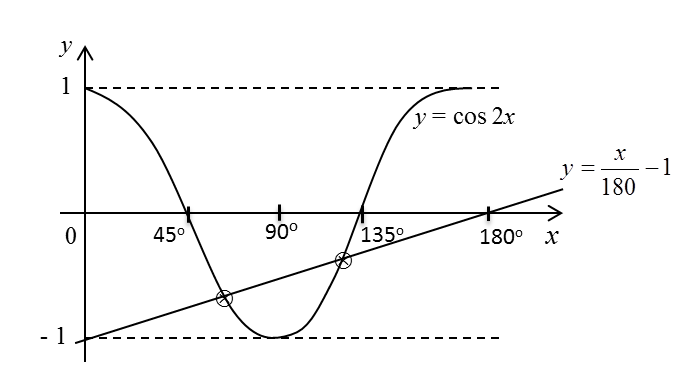# 6.8.1 Trigonometric Functions Long Questions (Question 1 & 2)

Question 1:
(a) Sketch the graph of y = cos 2x for 0°  x  180°.
(b) Hence, by drawing a suitable straight line on the same axes, find the number of solutions satisfying the equation for 0°  x  180°.

Solution:

(a)(b)Question 2:
(a) Prove that $\frac{2\mathrm{tan}x}{2-{\mathrm{sec}}^{2}x}=\mathrm{tan}2x.$
(b)(i) Sketch the graph of y = – tan 2x for 0  x ≤ π .
(b)(ii) Hence, by drawing a suitable straight line on the same axes, find the number of solutions satisfying the equation $\frac{3x}{\pi }+\frac{2\mathrm{tan}x}{2-{\mathrm{sec}}^{2}x}=0$  for 0  x π .
State the number of solutions.

Solution:
(a)
$\begin{array}{l}\frac{2\mathrm{tan}x}{2-{\mathrm{sec}}^{2}x}=\mathrm{tan}2x\\ \\ LHS:\\ \frac{2\mathrm{tan}x}{2-{\mathrm{sec}}^{2}x}=\frac{2\mathrm{tan}x}{2-\left(1+{\mathrm{tan}}^{2}x\right)}\\ \text{}=\frac{2\mathrm{tan}x}{2-{\mathrm{tan}}^{2}x}\\ \text{}=\mathrm{tan}2x\text{(RHS)}\end{array}$

(b)(i)(b)(ii)

When x = 0, y = 0.
When x = π, = 3.
Number of solutions = 3